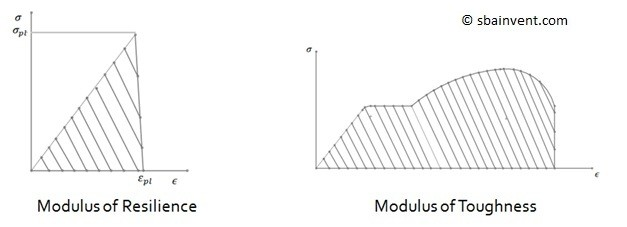# Energy

In order to deform a part energy is required. So how does this relate to Hook’s Law? The answer to this question comes from calculus. The energy to deform a part is the integral of the stress-strain curve used to determine Young’s Modulus. Now we already know what the equation is for the elastic region of stress strain curve.

(Eq 1)  $σ=Eε$

σ = stress

ε = strain

E = Young’s Modulus

So to find the total amount of energy the material will be able to resist before it starts to plastically deform the integral of the above equation would be taken. See below.

(Eq 2)  $u=∫\frac{σ}{E}dσ=\frac{1}{2}\frac{σ^2}{E}$

Now to make thing simple, an integral represent the area under the line of the equation used to find that integral. So this would mean that since the elastic region is linear, the area equation for a triangle can be used to find the energy that the material can absorb before it plastically deforms. See the equation below.

(Eq 3)  $u=\frac{1}{2}σε$

The total energy that a material can absorb under its elastic region is known as the modulus of resilience. However, looking at the stress strain curve it can be seen that there is additional energy that the material can absorb before catastrophic failure occurs. The total energy that the material can absorb before catastrophic failure is called the modulus of Toughness.